# Huffman Encoding

Updated:

Huffman codes compress data very effectively: savings of 20% to 90% are typical, depending on the characteristics of the data being compressed. We consider the data to be a sequence of characters. Huffman’s greedy algorithm uses a table giving how often each character occurs (i.e., its frequency) to build up an optimal way of representing each character as a binary string. —— CRLS

## 1. Encode and Decode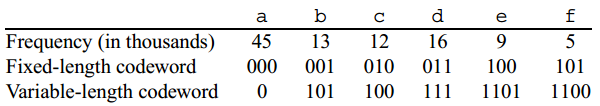### 1.1 Binary character code

Each character is represented by a unique binary string, which we call a codeword.

### 1.2 Fixed-/variable-length codeword

Say, a data file of 100,000 characters contains only the characters a~f, with the frequencies indicated.

• If we use a fixed-length code, we need 3 bits to represent 6 characters: a = 000, b = 001, . . . , f = 101. This method requires 300,000 bits to code the entire file.
• Using the variable-length code shown, we can encode the file in only 224,000 bits.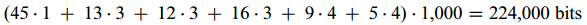// this method achieved a saving of approximately 25% and in fact, this is an optimal character code for this file

### 1.3 Prefix codes

(As the naming issue mentioned in CLRS, I do reckon that something like “prefix-free codes” might be a better name…)

A prefix code can always achieve the optimal data compression among any character code. —— CRLS

• Encoding is always simple for any binary character code; we just concatenate the codewords representing each character of the file.

• we code the 3-character file: “abc” as 0.101.100 = 0101100, where “.” denotes concatenation.
• Prefix codes are desirable because they simplify decoding ! Since no codeword is a prefix of any other, the codeword that begins an encoded file is unambiguous.

• By unambiguous, I mean we can simply identify the initial codeword, translate it back to the original character, and repeat the decoding process on the remainder of the encoded file.
• the string 001011101 parses uniquely as 0.0.101.1101, which decodes to "aabe".

### 1.4 Decoding representation

The decoding process needs a convenient representation for the prefix code so that we can easily pick off the initial codeword. A binary tree whose leaves are the given characters provides one such representation. —— CRLS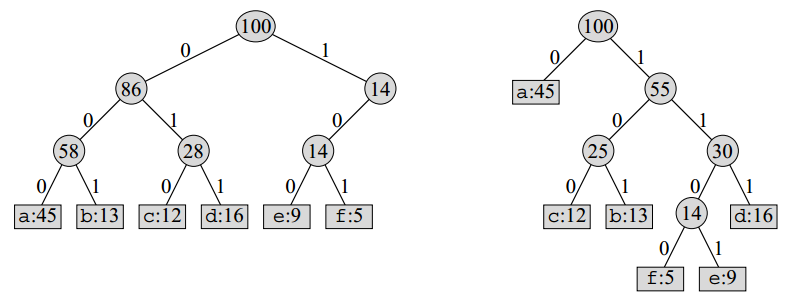#### Interpretation:

• A simple path from the root to a character: a codeword for a character
• 0: go to the left child
• 1: go to the right child

Tip: note that these trees are not BSTs as their leaves need not appear in sorted order, and internal nodes do not contain character keys.

### 1.5 Optimal code properties

An optimal code for a file is always represented by a full binary tree, in which every non-leaf node has two children. —— CRLS

Therefore, the fixed-length code in the figure is not optimal since its tree is not a full binary tree: it contains codewords beginning with 10. . ., but not beginning with 11. . ..

#### Binary tree (BT) properties for prefix coding:

• BT must be full;

• If C is the alphabet from which the characters are drawn, all character frequencies are positive;

• The tree for an optimal prefix code has exactly |C| leaves, one for each letter of the alphabet;

• The tree has exactly |C| - 1 internal nodes;

• Given a tree T corresponding to a prefix code, we can easily compute the number of bits required to encode a file as follows

• let’s denote c as each character in the alphabet C;
• c.freq denotes the frequency of c in a file;
• d_T(c) denotes the depth of the c’s leaf in T; ( Note that d_T(c) is also the length of the codeword for character c )
• The number of bits required to encode a file is thus ( the cost of the tree ):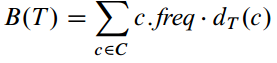## 2. Huffman encoding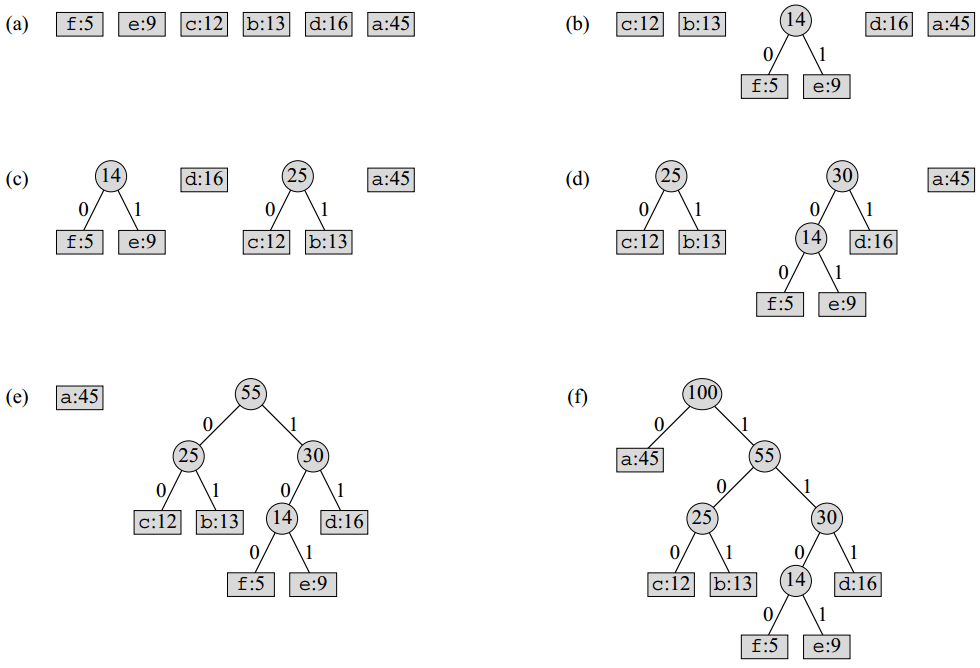### 2.1 Pseudocode

struct CharNode {
char value;
int  freq;

CharNode* left;
CharNode* right;
};

C = Set[CharNode]

Algorithm HuffmanEncoding(C):
n := |C|
Q := new MinPriorityQueue( BuildMinHeap(C, key=freq) )

For i := 1 to (n-1) do
newNode := new CharNode

newNode.left := ExtractMin(Q)
newNode.right:= ExtractMin(Q)
newNode.freq := newNode.left.freq + newNode.right.freq

MinHeapInsert(Q, newNode)

return ExtractMin(Q) --> return the root of the tree

• Huffman encoding builds a tree T corresponding to the optimal code in a bottom-up manner. It begins with a set of |C| leaves and performs a sequence of |C| - 1merging” operations to create the final tree. (n-1 times merging).

• The algorithm uses a min-priority queue Q keyed on the freq attribute in order to to identify the two least-frequent node objects to merge together.

• When we merge two nodes (setting the left and right for the newNode), the result is a new node whose frequency is the sum of the frequencies of the two nodes that were merged.

### 2.2 Time Complexity

• BuildMinHeap() in $O(n)$ time;
• The for-loop has (n-1) iterations;
• ExtractMin() and MinHeapInsert() in $O(lgn)$ time carrying on by the for-loop (n-1) times; ( recall each heap modification operation is in $O(lgn)$ )
$T(n) = O(n)+\Theta(n\ lgn) \in O(n\ lgn)$

Tips: We can reduce the running time to $O(nlglgn)$ by replacing the binary min-heap with a van Emde Boas tree (see CRLS Chapter 20).

Tags: